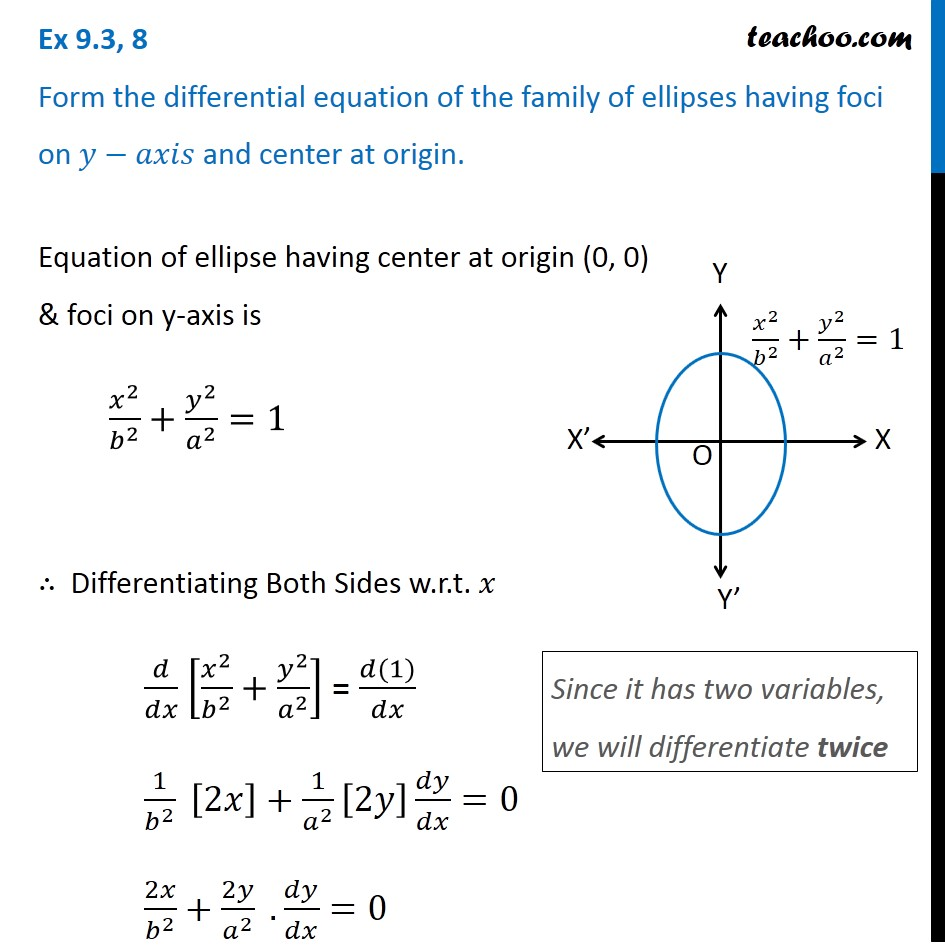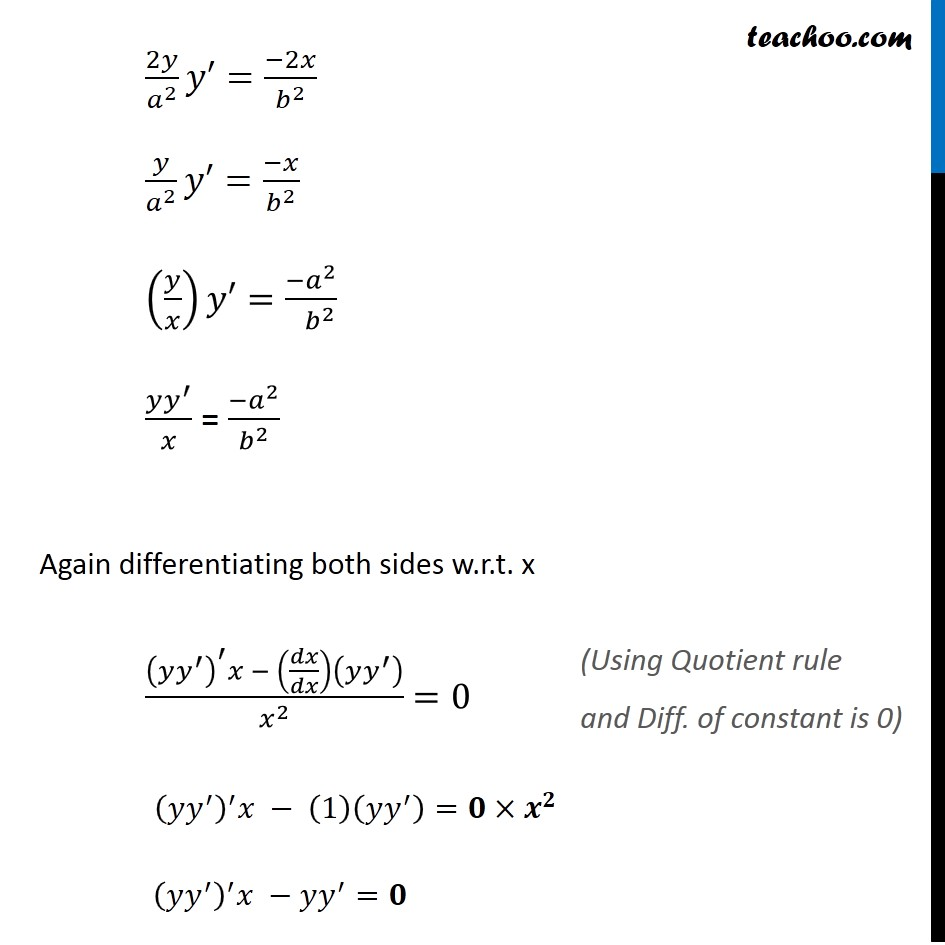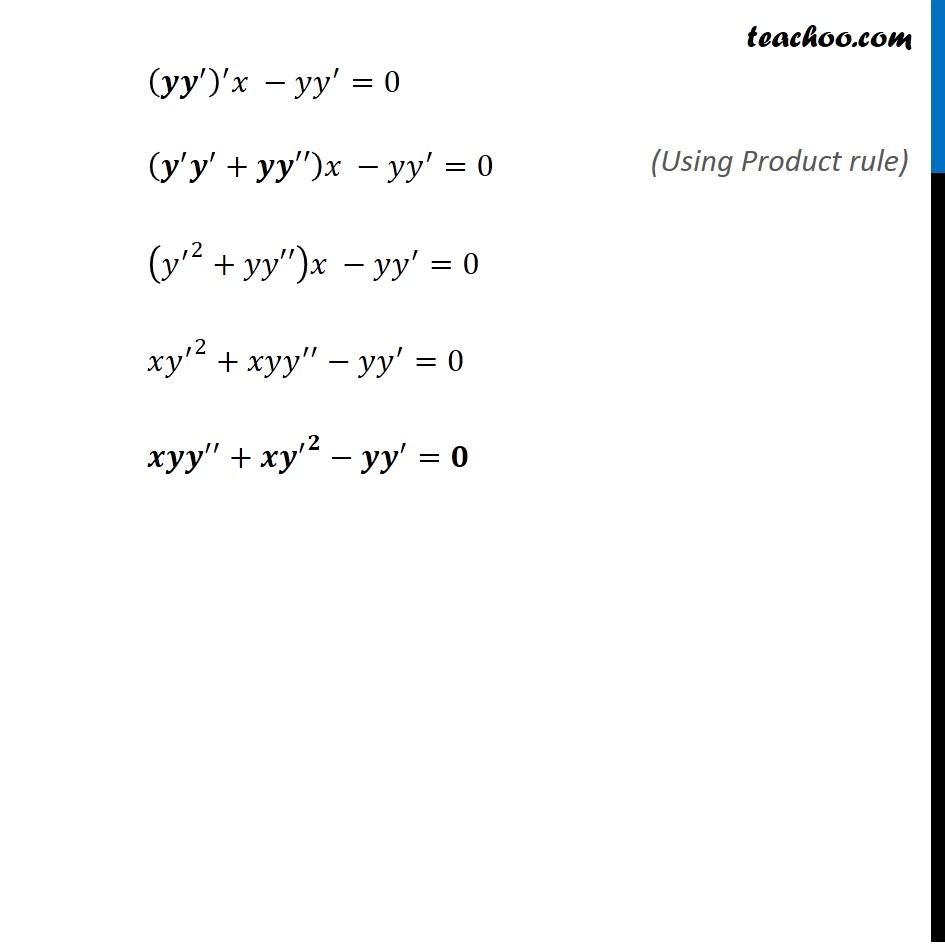Forming Differential equations

Chapter 9 Class 12 Differential Equations
Serial order wiseLearn in your speed, with individual attention - Teachoo Maths 1-on-1 Class

### Transcript

Question 8 Form the differential equation of the family of ellipses having foci on 𝑦−𝑎𝑥𝑖𝑠 and center at origin. Equation of ellipse having center at origin (0, 0) & foci on y-axis is 𝑥^2/𝑏^2 +𝑦^2/𝑎^2 =1 ∴ Differentiating Both Sides w.r.t. 𝑥 𝑑/𝑑𝑥 [𝑥^2/𝑏^2 +𝑦^2/𝑎^2 ] = (𝑑(1))/𝑑𝑥 1/𝑏^2 [2𝑥]+1/𝑎^2 [2𝑦] 𝑑𝑦/𝑑𝑥=0 2𝑥/𝑏^2 +2𝑦/𝑎^2 . 𝑑𝑦/𝑑𝑥=0 Since it has two variables, we will differentiate twice 2𝑦/𝑎^2 𝑦′=(−2𝑥)/𝑏^2 𝑦/𝑎^2 𝑦′=(−𝑥)/𝑏^2 (𝑦/𝑥)𝑦′=(−𝑎^2)/〖 𝑏〗^2 (𝑦𝑦^′)/𝑥 = (−𝑎^2)/𝑏^2 Again differentiating both sides w.r.t. x ((𝑦𝑦^′ )^′ 𝑥 − (𝑑𝑥/𝑑𝑥)(𝑦𝑦^′ ))/𝑥^2 =0 (𝑦𝑦^′ )^′ 𝑥 − (1)(𝑦𝑦^′ )=𝟎×𝒙^𝟐 (𝑦𝑦^′ )^′ 𝑥 −𝑦𝑦^′=𝟎 (Using Quotient rule and Diff. of constant is 0) (𝒚𝒚^′ )^′ 𝑥 −𝑦𝑦^′=0 (𝒚^′ 𝒚^′+𝒚𝒚′′)𝑥 −𝑦𝑦^′=0 (〖𝑦^′〗^2+𝑦𝑦′′)𝑥 −𝑦𝑦^′=0 𝑥〖𝑦^′〗^2+𝑥𝑦𝑦^′′−𝑦𝑦^′=0 𝒙𝒚𝒚^′′+𝒙〖𝒚^′〗^𝟐−𝒚𝒚^′=𝟎 (Using Product rule)